Get instant live expert help with Excel or Google Sheets“My Excelchat expert helped me in less than 20 minutes, saving me what would have been 5 hours of work!”

#### Post your problem and you’ll get Expert help in seconds.

Your message must be at least 40 characters
Our professional Expert are available now. Your privacy is guaranteed.

# Get first day of previous month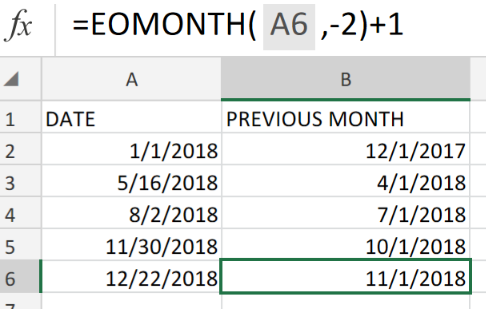Figure 1. Get First Day of Previous Month in Excel.

If we are required to get the first day of a previous month in Excel, we are going to utilize the EOMONTH function.

## Generic Formula

`=EOMONTH(date,-2)+1`

The EOMONTH function operates by calculating the amount of days and months that it must roll back before determining the first day of the previous month.

## How to use the Excel EOMONTH function.

The EOMONTH function operates by calculating the amount of days and months that it must roll back before determining the first day of the previous month. It starts by rolling back 2 months, then proceeds to add 1 day to that particular date which gives us the first day occurring in the previous month.

We are going to demonstrate how we can use the Excel EOMONTH function in 3 simple steps;

1. Arrange the dates we are going to check out in a labeled column of our worksheet. Be sure to make provision for the empty cells where Excel will return our results.

See example illustrated below;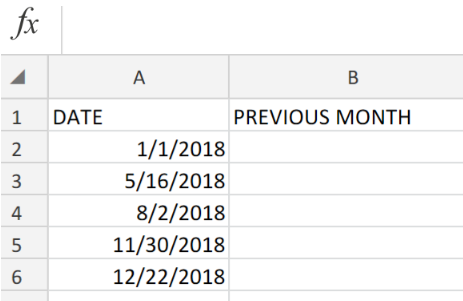Figure 2. Get The First Day of Previous Month in Excel.

1. In our example above, our EOMONTH formula to be entered into cell B2 is as follows;

`=EOMONTH(A2,-2)+1`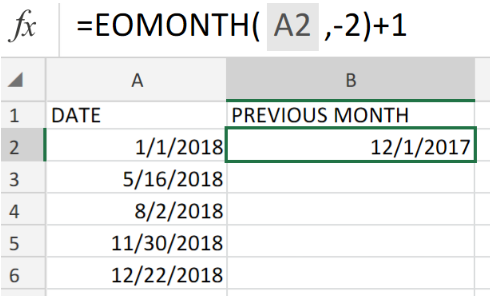Figure 3. Get The First Day of Previous Month in Excel.

1. Excel returned the first date result as 12/1/2017. In order to get the other dates, copy a modified version of the EOMONTH formula in cell B2 then enter into the other cells in the PREVIOUS MONTH column.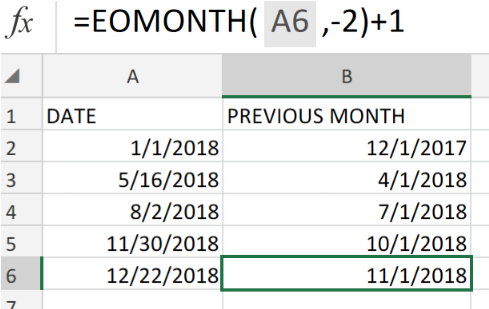Figure 4. Get The First Day of Previous Month in Excel.

The last day of a month based on a given date can be determined by the Excel EOMONTH function.

We can calculate the first day of the previous month from any given date traveling back 2 months, then adding one day.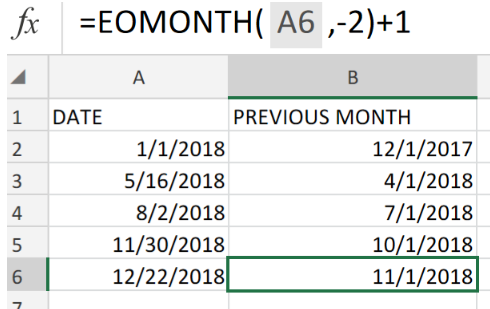Figure 5. Final Result.

## Instant Connection to an Expert through our Excelchat Service:

Our live Excelchat Service is here for you. We have Excel Experts available 24/7 to answer any Excel questions you may have. Guaranteed connection within 30 seconds and a customized solution for you within 20 minutes

### Did this post not answer your question? Get a solution from connecting with the expert.Another blog reader asked this question today on Excelchat:
Solution exampleshow do I derive the monthly sales from a date and revenue column
Solved by A. U. in 23 minsI need to sum the number of hours per month one of three halls are being rented
Solved by A. F. in 60 minsI can't get this formula correct, can someone assist? I am trying to create a date for our posting process based on the payment terms. If a customer is paying biweekly, with a start date of 3/1/2018, etc. I want to be able to run daily what customers will need to be charged. =IF(OR(D2="MONTHLY",\$C\$6+30,")*IF(D2="WEEKLY",\$C\$6+7,"")*IF(D2="BIWEEKLY",\$C\$6+14,""""))
Solved by S. H. in 9 minsHOW CAN I COUNT NUMBERS PER DAY FOR A MONTH AS TOTAL?
Solved by F. F. in 16 minsa date formula that results in showing the date as 29/12/00 (date format as dd/mm/yy) - use column BA to calculate 364 days (same date format) in column BS, if BA blank, then calculate 364 days using column AX. Results for column BA are correct. However, if column BA is empty, then it should calculate using column AX, which has data, but the result is always 29/12/00, regardless of the date in column AX. I have used this formula with success in another workbook, but this file wont work! Formula: =IF(AND(LEN(\$BA74)=0,LEN(\$AX74)=0),"",IF(LEN(\$BA74)=0,DATE(YEAR(\$AX74),MONTH(\$AX74),DAY(\$AX74)+364),DATE(YEAR(\$BA74),MONTH(\$BA74),DAY(\$BA74)+364)))
Solved by A. L. in 60 mins## Subscribe to Excelchat.coAnother blog reader asked this question today on Excelchat: